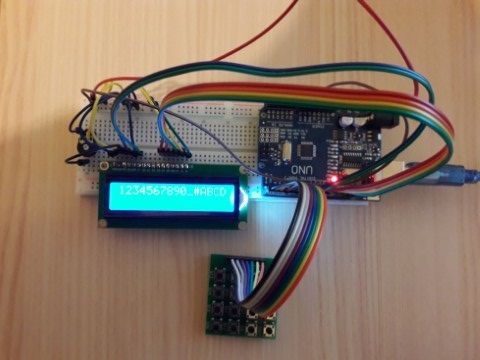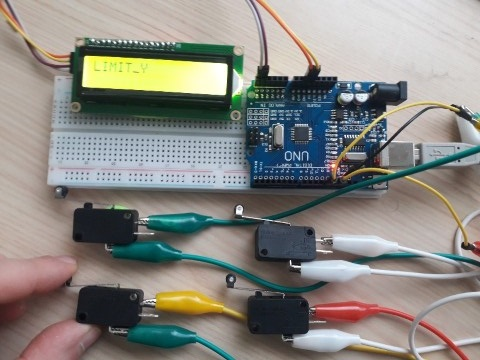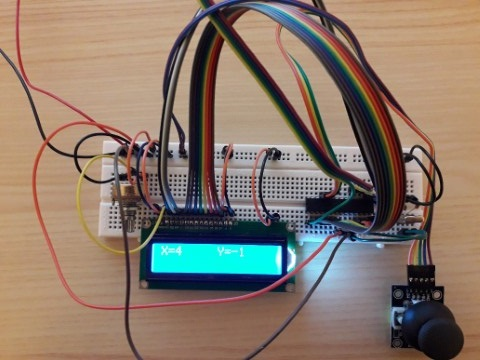# Input devices

## keypad with LCD display (4bit mode) with arduino

#include libraries
/// initialize the library with the numbers of the interface pins

LiquidCrystal lcd(8, 9, 10, 11, 12, 13);//RS,EN,D4,D5,D6,D7

const byte ROWS = 4; // Four rows

const byte COLS = 4; // Three columns

// Define the Keymap

char keys[ROWS][COLS] = {

{'1','5','9','A'},

{'2','6','0','B'},

{'3','7','_','C'},

{'4','8','#','D'}

};

// Connect keypad ROW0, ROW1, ROW2 and ROW3 to these Arduino pins.

byte rowPins[ROWS] = { 0, 1, 2, 3 };

// Connect keypad COL0, COL1 and COL2 to these Arduino pins.

byte colPins[COLS] = { 4, 5, 6, 7 };

void setup()

{

for(int k=8;k<14;k++)

{
pinMode(k,OUTPUT);//pins 8-14 are enabled as output
}
lcd.begin(16, 2);//initializing LCD
}
void loop()
{
char key = kpd.getKey(); //storing pressed key value in a char

if (key != NO_KEY)
{
lcd.print(key); //showing pressed character on LCD
}
}### Limit-switch with LCD display (+I2C module) with arduino

#include libraries
// Set the LCD address to 0x3F for a 16 chars and 2 line display
LiquidCrystal_I2C lcd(0x3F,16,2);

const int LIMIT_PIN_X = 13; // the number of the x limit pin
const int LIMIT_PIN_Y = 12; // the number of the y limit pin
int LIMIT_STATE_X = 1; // variable for reading the x lmit status
int LIMIT_STATE_Y = 1; // variable for reading the y limit status

void setup()
{
pinMode(LIMIT_PIN_X, INPUT_PULLUP); // define the limit switch pin as input with pullup res
pinMode(LIMIT_PIN_Y, INPUT_PULLUP);
lcd.init();
lcd.backlight();

}
void loop()
{

lcd.setCursor(0, 0);

LIMIT_STATE_X = digitalRead(LIMIT_PIN_X); // read the state of the x limit pin
LIMIT_STATE_Y = digitalRead(LIMIT_PIN_Y); // read the state of the y limit pin

if (LIMIT_STATE_X == HIGH) { // if the x pin is high
lcd.print("LIMIT_X"); }

else if (LIMIT_STATE_Y == HIGH) { // if the y pin is hign
lcd.print("LIMIT_Y"); }

else {
lcd.clear();
}

}### Joystick with LCD display (8 bit mode) on Bread-board with C code

#include libraries
#define lcd_data PORTD //LCD data port
#define ctrl PORTB #define en PB2 // enable signal
#define rw PB1 // read or write signal
#define rs PB0 // register select signal

//------LCD-----------

void lcd_cmd(unsigned char cmd);
void init_lcd(void);
void lcd_write(unsigned char data);

//-------Main-------

int main(void)
{
char buffer;

DDRD=0xff;
DDRB=0x07;

LCD_init(); /* Initialize LCD */

_delay_ms(50); // delay of 50ms
lcd_write_string("HELLO");// prints string on LCD
_delay_ms(1000); // delay of 50ms
lcd_cmd(0x01); // clear LCD
_delay_ms(1);

while(1)
{
lcd_cmd(0x80); // ---8 go to first line and --0 is for 0th position
lcd_write_string(buffer);

lcd_cmd(0x88);
lcd_write_string(buffer);

{
lcd_cmd(0x01); // clear LCD
lcd_cmd(0x0E); // cursor ON
lcd_cmd(0x80); // ---8 go to first line and --0 is for 0th position
lcd_write_string("Switch pressed");
_delay_ms(1000); // delay of 50ms
lcd_cmd(0x01); // clear LCD
lcd_cmd(0x0E); // cursor ON
}
}
}

//--------LCD-----------------

void LCD_init(void)
{
lcd_cmd(0x38); // LCD initialization
_delay_ms(1);

lcd_cmd(0x01); // clear LCD
_delay_ms(1);

lcd_cmd(0x0E); // cursor ON
_delay_ms(1);

lcd_cmd(0x80); // ---8 go to first line and --0 is for 0th position
_delay_ms(1);
return;
}

void lcd_cmd(unsigned char cmd)
{
lcd_data=cmd;
ctrl =(0< _delay_ms(1);
ctrl =(0< _delay_ms(50);
return;
}

void lcd_write(unsigned char data)
{
lcd_data= data;
ctrl = (1< _delay_ms(1);
ctrl = (1< _delay_ms(50);
return ;
}

void lcd_write_string(unsigned char *str)//store address value of the string in pointer *str
{
int i=0;
while(str[i]!='\0')// loop will go on till the NULL character in the string
{
lcd_write(str[i]);// sending data on LCD byte by byte
i++;
}
return;
}

{